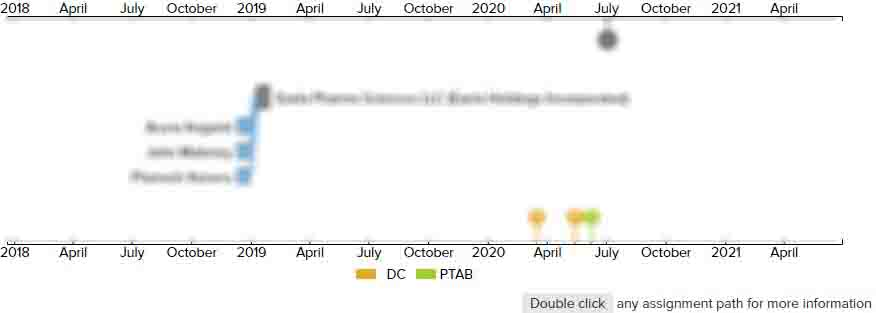# Modulation and demodulation techniques for a health monitoring system

• US 10,219,754 B1
• Filed: 02/12/2015
• Issued: 03/05/2019
• Est. Priority Date: 09/09/2014
• Status: Active Grant
##### First Claim
Patent Images

1. A method for estimating physiological parameters when modulated light from a first light source and a second light source is emitted toward a body part of a user, the method comprising:

• determining a first multiplier value by;

turning on the first light source;

generating a first initial signal in response to capturing a first light sample corresponding to the first light source;

demodulating the first initial signal to produce first initial demodulated signals;

filtering and decimating the first initial demodulated signals; and

determining the first multiplier value based on the filtered and decimated first initial demodulated signals;

determining a second multiplier value by;

turning on the second light source;

generating a second initial signal in response to capturing a second light sample corresponding to the second light source;

demodulating the second initial signal to produce second initial demodulated signals;

filtering and decimating the second initial demodulated signals; and

determining the second multiplier value based on the filtered and decimated second initial demodulated signals;

capturing multiple light samples while the first light source and the second light source are turned on to emit modulated light toward the body part of the user and converting the multiple light samples into a captured signal;

demodulating the captured signal to produce multiple demodulated signals;

performing a first decimation stage by;

low pass filtering each demodulated signal; and

decimating each demodulated signal;

performing a second decimation stage after the first decimation stage by;

low pass filtering each demodulated signal; and

decimating each demodulated signal;

demultiplexing each demodulated signal after the second decimation stage to produce a first signal associated with the first light source and a second signal associated with the second light source;

multiplying the first signal by the first multiplier value using a first multiplier circuit to obtain a first conditioned signal;

multiplying the second signal by the second multiplier value using a second multiplier circuit to obtain a second conditioned signal; and

analyzing the first conditioned signal and the second conditioned signal to estimate the physiological parameter of the user.

• ##### 1 Assignment
Timeline View
Assignment View×
×